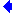Search IntMath
Close

# Rule of 72

There is a curious and helpful trick that allows us to mentally estimate annual compound interest amounts, where we are interested in doubling our money.

The Rule of 72 works as follows.

If we want to know how long it will take for our money to double, just divide 72 by the interest rate.

So for example, if the interest rate is 10%,

72 ÷ 10 = 7.2 years

So it will take just over 7 years to double our money.

If the interest rate is 8%, to double our money it will take

72 ÷ 8 = 9 years

Here is a graph showing $1 doubling at different interest rates. The growth of A=$1 at 3% (the slowest growing), 5%, 8%, 10% and 12% (the fastest growth), showing (with a pink dot) when it doubles to value $2. #### Finding Interest We can use the Rule of 72 the other way around too. Say we have a 15 year time span and we want to double our money in that time. What interest rate do we need so that the money will double? Answer: 72 ÷ 15 = 4.8% ### How Does Rule of 72 Work? From the last section (Interesting Interest), the amount of money we have after investing P dollars for t years at r% interest (as a decimal) is given by: A = P(1 + r)t We want to know how long it takes to double our$A to \$2A.

2A = A(1 + r)t

Cancelling gives:

2 = (1 + r)t

Using logarithms to solve this equation, we have (recall ln means log_e):

ln 2 = t ln(1 + r)

t=(ln\ 2)/(ln(1+r))

We can find the value of the right hand side for different values of r. When we multiply these values by r, an interesting thing occurs − the values are very near 72.

#### Example

If r = 3% = 0.03, then:

t=(ln\ 2)/(ln(1+r))=(ln\ 2)/(ln (1.03))=23.45

This means it would take more than 23 years to double our money at an interest rate of 3%.

Now multiplying 23.45 by 3, we find

23.45 xx 3 = 70.35.

We see that this (value of years) xx (interest rate) is quite close to 72.

### Range of interest rates

Let’s now do the same for a range of typical interest rates from r = 2 through to r = 14.

We get:

 Rate Years Rate xx Years 2% 35.00 70.01 3% 23.45 70.35 4% 17.67 70.69 5% 14.21 71.03 6% 11.90 71.37 7% 10.24 71.71 8% 9.01 72.05 9% 8.04 72.39 10% 7.27 72.72 11% 6.64 73.06 12% 6.12 73.40 13% 5.67 73.73 14% 5.29 74.06
(Rounded to 2 decimal places)

We observe that the values in the last column are near 72. So we can approximate t (the time it takes to double our money for a given interest rate, r) as:

t~~72/r

Equivalently, we can approximate r (the interest rate needed to double our money for a given interest time, t) as:

r~~72/t

Here is a graph of the curve r=72/t with the values given in the above table (the pink dots). We can see the curve very closely approximates the situation for a wide range of interest rates.

The graph of r=72/t (our approximation), with the actual times for doubling our money for various interest rates from the table above.

### Conclusion

The Rule of 72 gives us an easy "back of the envelope" calculation for the time it will take to double our money. It is good for a range of typical interest rates, from 5% to about 12%. Even for high interest rates like 20%, the value is 76.04.

If you are interested, go back toInteresting Interest.

## Problem SolverThis tool combines the power of mathematical computation engine that excels at solving mathematical formulas with the power of GPT large language models to parse and generate natural language. This creates math problem solver thats more accurate than ChatGPT, more flexible than a calculator, and faster answers than a human tutor. Learn More.